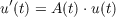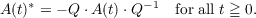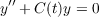#### Vol. 16, No. 3, 1966

 Download this articleFor screen For printingRecent Issues Vol. 325: 1 Vol. 324: 1  2 Vol. 323: 1  2 Vol. 322: 1  2 Vol. 321: 1  2 Vol. 320: 1  2 Vol. 319: 1  2 Vol. 318: 1  2Online Archive Volume: Issue:The Journal Subscriptions Editorial Board Officers Contacts Submission Guidelines Submission Form Policies for Authors ISSN: 1945-5844 (e-only) ISSN: 0030-8730 (print) Special Issues Author Index To Appear Other MSP Journals
Stability of linear differential equations with periodic coefficients in Hilbert space

### Gert Einar Torsten Almkvist

Vol. 16 (1966), No. 3, 383–391
##### Abstract

In this paper we study the stability of the solutions of the differential equation(1)

for t 0 in a separable Hilbert space. It is assumed that A(t) is periodic with period one and satisfies the following symmetry condition: There exists a continuous constant invertible operator Q such thatWe use a perturbation technique. Let A(t) = A0(t) + B(t) where A0(t) is compact and antihermitian for all t. We denote by U0(t) the solution operator of u(t) = A0(t)u(t). It is shown that (1) is stable if B(t) satisfies a certain smallness condition involving the distribution of the eigenvalues of U0(1) and the action of B(t) on the eigenvectors of U0(1). The results can be applied to the second order equationwhere C(t) is selfadjoint for all t.

Primary: 34.95
Secondary: 34.51
##### Milestones